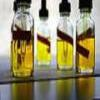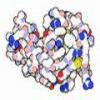#### You may also like### Mathematical Issues for Chemists

A brief outline of the mathematical issues faced by chemistry students.### Reaction Rates

Explore the possibilities for reaction rates versus concentrations with this non-linear differential equation### Mixed up Mixture

Can you fill in the mixed up numbers in this dilution calculation?

# Heavy Hydrocarbons

##### Age 14 to 18Challenge Level

This problem consists of a series of questions concerning hydrocarbons made from the stable isotopes of hydrogen and carbon. Assume that the probability of the occurrence of unstable isotopes is effectively zero. Assume also that the mass of an isotope is equal to the mass number.

Carbon is found in two naturally occurring stable isotopic forms: Carbon-12, $^{12}$ C, accounts for $98.9\%$ of naturally occurring carbon, and Carbon-13, $^{13}$ C, accounts for $1.1\%$ of naturally occurring carbon.

Hydrogen also has two stable isotopes: $^1$H accounts for slightly more than $99.985\%$ of naturally occurring hydrogen. $^2$H, called deuterium, accounts for about $0.015\%$ of naturally occurring hydrogen.

What possibilities are there for the molecular mass of a stable molecule of methane CH$_4$? Which three are the most common? What is the probability that a randomly encountered methane molecule will be of each of these masses?

What would be the three most likely possibilities for the molecular masses of the next two alkanes: ethane, C$_2$H$_6$, and propane C$_3$H$_8$?

Am I likely to find a molecule of butane C$_4$H$_{10}$ of molecular mass $72$ in a litre of butane? (Avogadro's constant is approximately $6.02\times 10^{23}$).

Are there any alkanes for which the most commonly found molecule is not the one made entirely from $^{12}$C and $^1$H?

NOTES AND BACKGROUND

Mass spectrometry allows chemists to determine the molecular masses of compounds. The frequency of occurrence of common isotopes of elements is an important factor in understanding such as analysis. Mass spectrometry is of great industrial and scientific importance, and in practice, the results of an analysis are determined by comparison with a database of experimental spectrometry information.

For information on alkanes and hydrocarbons, see http://en.wikipedia.org/wiki/Hydrocarbon